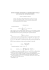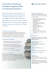# RQ 7 - Solutions

## Transcription

RQ 7 - Solutions
```UCSC
ECON/AMS 11A
Review Questions 7
Solutions
1. Find the critical points of the functions of the functions below, and classify their critical
values (as relative minimum, relative maximum, or neither) using either the first derivative
test or the second derivative test.
a. f (x) = x3 − 6x2 − 15x + 2
f 0 (x) = 3x2 − 12x − 15 = 3(x2 − 4x − 5) = 3(x − 5)(x + 1). Solving the equation
f 0 (x) = 0 gives the critical points x1 = −1 and x2 = 5.
f 00 (x) = 6x − 12, and f 00 (−1) = −18 < 0, so f (−1) = 10 is a relative maximum value,
by the second derivative test. Likewise, f 00 (5) = 18 > 0, so f (5) = −98 is a relative
minimum value.
b. g(t) = t4 e−t
7.5
Max Value
5
Min Value
2.5
0
2.5
5
7.5
10
12.5
15
Figure 1: Graph of the function from 1b.
g 0 (t) = 4t3 e−t − t4 e−t = t3 e−t (4 − t). Solving the equation g 0 (t) = 0 gives the critical
points t1 = 0 and t2 = 4.
g 00 (t) = 12t2 e−t − 4t3 e−t − 4t3 e−t − t4 e−t = t2 e−t t2 − 8t + 12 . Evaluating the
second derivative at the critical points, we have g 00 (0) = 0 and g 00 (4) = 16e−4 · (−4) < 0.
Thus, g(4) = 256e−4 ≈ 4.6888 is a relative maximum value, by the second derivative
test, but the same test is inconclusive with regards to g(0). This means that we have
to use the first derivative test for t1 = 0.
Evaluating g 0 (t) to the left and right of 0, we find that g 0 (−1) = −5e < 0, and g 0 (1) =
3e−1 > 0. This means that the function g(t) is decreasing to the left of 0 and increasing
to the right of 0, so g(0) = 0 is a relative minimum value.
√
63s
6s1/3
c. h(s) = 2
= 2
.
s +5
s +5
2s2 + 10
− 12s4/3
2/3
2s2 − 12s2 + 10
10(1 − s2 )
+ 5) −
s
0
=
=
=
.
h (s) =
(s2 + 5)2
(s2 + 5)2
s2/3 (s2 + 5)2
s2/3 (s2 + 5)2
Solving the equation h0 (s) = 0 produces 2 critical points, s1 = −1 and s2 = 1, but
that’s not the end of the story, because h0 (s) is undefined when s = 0, so s3 = 0 is also
a critical point. To classify the critical values I’ll use the first derivative test here, and to
make this easier, you should note that the denominator of h0 (s) is always positive when
s 6= 0, so the sign of the derivative will depend on the sign of the numerator of h0 (s).
With this observation, it is easy to see that
2s−2/3 (s2
h0 (−2) =
12s4/3
−30
7.5
7.5
−30
< 0, h0 (−0.5) =
> 0, h0 (0.5) =
> 0 and h0 (2) =
< 0.
(+)
(+)
(+)
(+)
This means that h(−1) = −1 is a relative minimum and h(1) = 1 is a relative maximum
value, and h(0) = 0 is neither a minimum value nor a maximum value, since the graph
does not change direction at 0.
2
Max Value
1.5
1
0.5
-6
-5
-4
-3
-2
-1
0
1
2
3
4
5
6
-0.5
-1
-1.5
Min Value
-2
Figure 2: Graph of the function from 1c.
2. For the functions g(t) and h(s) in problem 1. determine whether any of the relative extreme
values that you found are absolute extreme values on the interval (−∞, ∞). Justify your
a. g(0) = 0 is the absolute minimum value because g(t) = t4 e−t > 0 when t 6= 0. On
the other hand, g(4) ≈ 4.6888 is not the absolute maximum value of g(t) on (−∞, ∞),
since g(−10) = 10000e10 ≈ 220264657.9, for example, is bigger.
Note that g(4) is the absolute maximum value of g(t) on the interval (0, ∞), since t = 4
is the only critical point in (0, ∞), and we have already seen that g(4) is a relative
maximum value. (See the graph in Figure 1.)
b. The denominator of h(s), (s2 + 5), is always positive and the numerator of h(s), (6s1/3 ),
is negative for negative arguments and positive for positive arguments. This means that
when s > 0 then h(s) > 0 and when s < 0, then h(s) < 0. Now, the critical point s = 1
is the only critical point in the interval (0, ∞), so h(1) = 1 is the absolute maximum
value of h(s) for s > 0. But h(1) = 1 > 0 ≥ h(s) for all s ≤ 0, by the comment above.
So h(1) = 1 is the absolute maximum value of h(s) in (−∞, ∞).
Likewise, the critical point s = −1 is the only critical point in (−∞, 0), and since
h(−1) = −1 is a relative minimum, it follows that h(−1) = −1 is the absolute minimum
value of h(s) for s < 0. But, h(−1) = −1 < 0 ≤ h(s) for all s ≥ 0, according to
the argument above, so h(−1) = −1 is the absolute minimum value of h(s) in all of
(−∞, ∞). See the graph in Figure 2.
3. Find the inflection point(s) of the function f (x) in problem 1.
An inflection point is a point on the graph of a function where the concavity changes. To
find inflection points we look at the points where the second derivative of the function is
either 0 or undefined. In the case at hand, we have f (x) = x3 − 6x2 − 15x + 2, and
f 0 (x) = 32 − 12x − 15, so f 00 (x) = 6x − 12. The second derivative is linear, and hence it
is defined for all x, so the only candidate for an inflection point is the point (2, −44), since
f 00 (2) = 0. Now, if x < 2, then f 00 (x) = 6x − 12 < 6 · 2 − 12 < 0, and similarly, if x > 2,
then f 00 (x) > 0. This means that the graph y = f (x) is concave down when x < 2 and
y = f (x) is concave up when x > 2. So the concavity changes at (2, −44), which means
that this is indeed an inflection point.
4. Find the absolute maximum and absolute minimum values of the function f (x) = x4 −8x2 +1
on the interval [−1, 5].
First we find the critical points of the function in the given interval,
f 0 (x) = 4x3 − 16x = 4x(x2 − 4) = 4x(x − 2)(x + 2),
so the critical points for this function are x0 = 0, x1 = −2 and x2 = 2. Of these three
critical points, only x0 = 0 and x2 = 2 are relevant, since x1 = −2 is not in the interval
[−1, 5].
Next, since this is a closed interval, we need only evaluate the original function f (x) at the
critical points and at the endpoints of the interval to locate the absolute max and min value.
We have
f (−1) = −6,
f (0) = 1,
f (2) = −15,
f (5) = 426,
so the minimum value of f (x) is −15 and the maximum value of f (x) is 426.
5. Show that the equation x2 − 8 ln x = 0 has exactly two real solutions.
8
. Setting f 0 (x) = 0 yields the solutions
x
x = ±2, but since f (x) is only defined on (0, ∞), there is only one critical point: x0 = 2.
If we set f (x) = x2 − 8 ln x, then f 0 (x) = 2x −
10
> 0, so f (2) ≈ −1.545 < 0 is a relative minimum
3
value. Since x0 = 2 is the only critical point, it follows that f (x) is decreasing between 0
and 2, and f (x) is increasing between 2 and ∞.†
Now, f 0 (1) = −6 < 0 and f (3) =
This means, in particular, that the graph of f (x) can cross the x-axis at most once between
0 and 2, and at most once between 2 and ∞. Since f (1) = 1 > 0 and f (3) ≈ 0.211 > 0,
it follows that in fact, f (x) = 0 exactly twice—once in the interval (1, 2) and once in the
interval (2, 3).
20
15
10
5
0
0.4
0.8
1.2
1.6
2
2.4
2.8
3.2
3.6
4
4.4
4.8
Figure 3: Graph of y = x2 − 8 ln x.
†
It also follows that f (2) is the absolute minimum value of f (x) on (0, ∞), but that is not directly
relevant to the question at hand.
```

### CALCULUS I Worksheet #69 1. Find all inflection points for f(x) = 2x3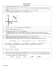### AP Calculus AB – Worksheet 83 The Second Derivative and The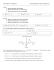### Math 220 Calculus I Exam 2 Fall 13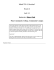### Summary of the rules of differentiation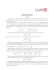### Document 6576713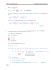### Week 6 notes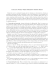### Homework 10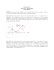### Name: AP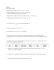### Worksheet #15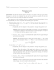### MATH 130 HW Problems Key Exam 2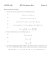### Small-Sample C.I.s for one- sample, two-sample and (matched) paired data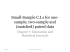### MATH 121, Calculus I — Sample Exam I (Fall 2013) Name: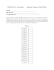### SAMPLE MEANS 8/4/2014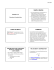### Aggregate Project Planning Methodology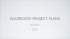### HW3 out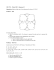### Sophisticated Graphing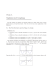### SPINORS ON THE RIEMANNIAN MANIFOLDS 1. Introduction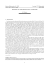### MITTAG-LEFFLER FUNCTIONS, LP GENERALIZED N-BALLS